## 第十四、十五周作业

1、实验代码

#include <stdio.h>
int main(void){
int i,sum=0;
int a;
for(i=0;i<10;i++){
scanf("%d",&a[i]);
}
for(i=0;i<10;i++){
if(a[i]%2==0)
sum=sum+a[i];
}
printf("s=%d",sum);
return 0;
}

2、设计思路

（1）主要描述

（2）流程图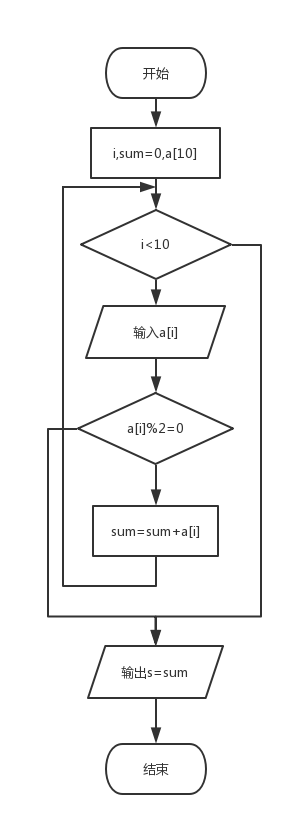3、本题调试过程碰到的问题及解决办法

提示截图：1、实验代码

#include <stdio.h>
int main(void)
{
int i,index,n;
printf("Enter n:");
scanf("%d",&n);
int a[n];
printf("Enter %d integers:",n);
for(i=0;i<n;i++)
scanf("%d",&a[i]);
index=0;
for(i=0;i<n;i++)
if(a[i]>a[index])
index=i;
printf("max is %d\n index is %d\n",a[index],index);
return 0;
}

2、设计思路

（1）主要描述

（2）流程图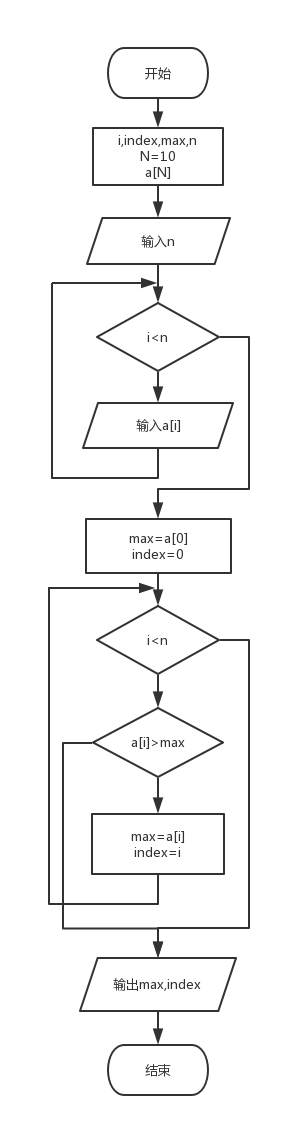3、本题调试过程碰到的问题及解决办法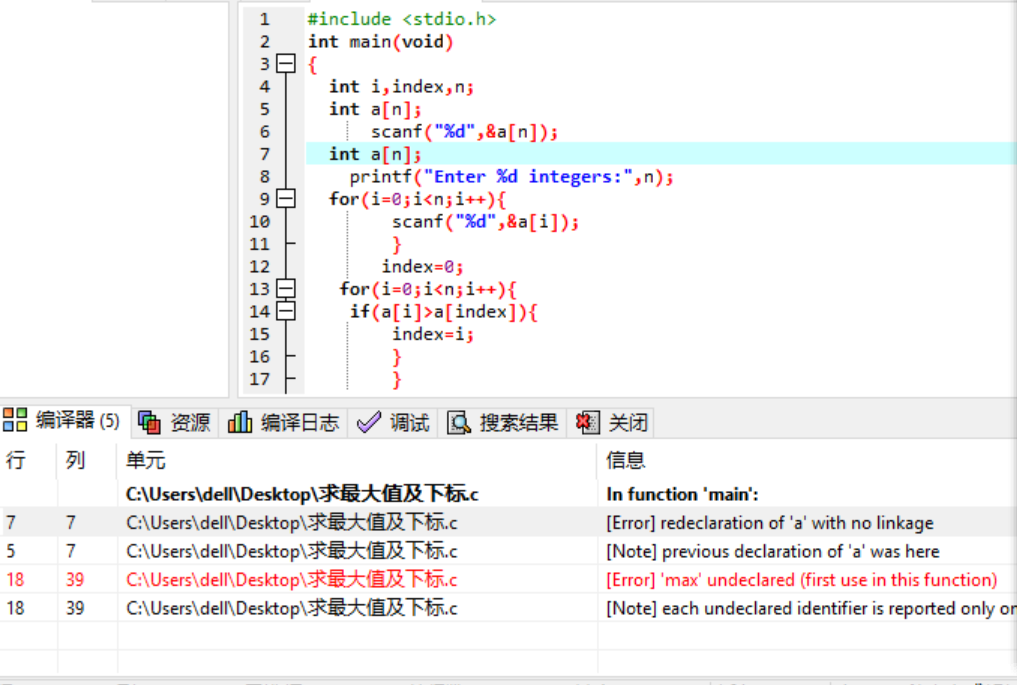1、实验代码

#include <stdio.h>
int main(void)
{
int i,j,sum=0;
int a;
for(i=0;i<4;i++)
{
for(j=0;j<4;j++)
{
scanf("%d ",&a[i][j]);
if(j<=i)
{sum=sum+a[i][j];}
}
}
printf("%d",sum);
return 0;
}

2、设计思路

（1）主要描述

（2）流程图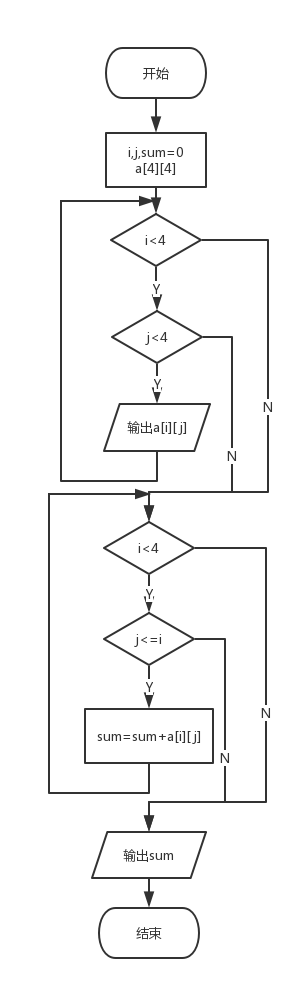3、本题调试过程碰到的问题及解决办法1、实验代码

#include <stdio.h>
int main(void){
int i;
int fib={1,1};
printf("%6d",fib);
printf("%6d",fib);
for(i=2;i<12;i++){
fib[i]=fib[i-1] + fib[i-2];
printf("%6d",fib[i]);
if((i+1)%3 == 0){
printf("%c",'\n');
}
}
return 0;
}

2、设计思路

（1）主要描述

（2）流程图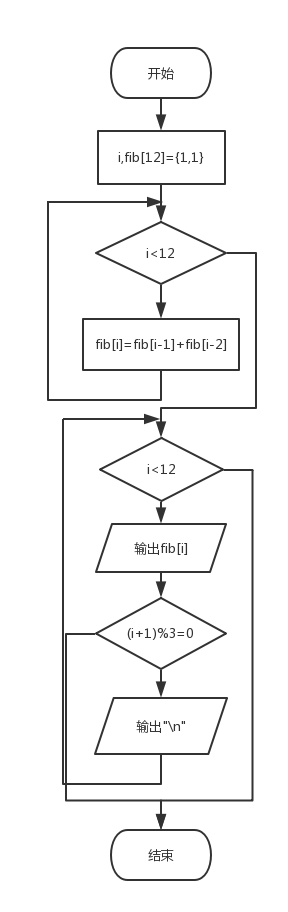3、本题调试过程碰到的问题及解决办法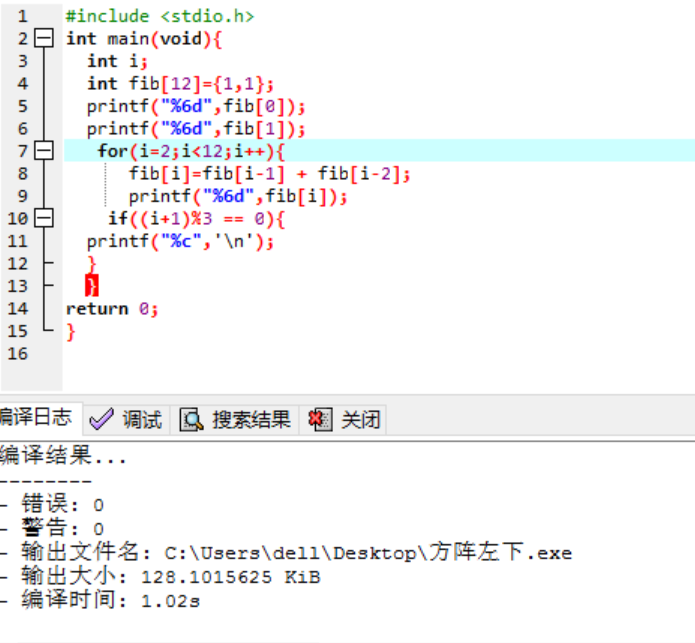提交列表：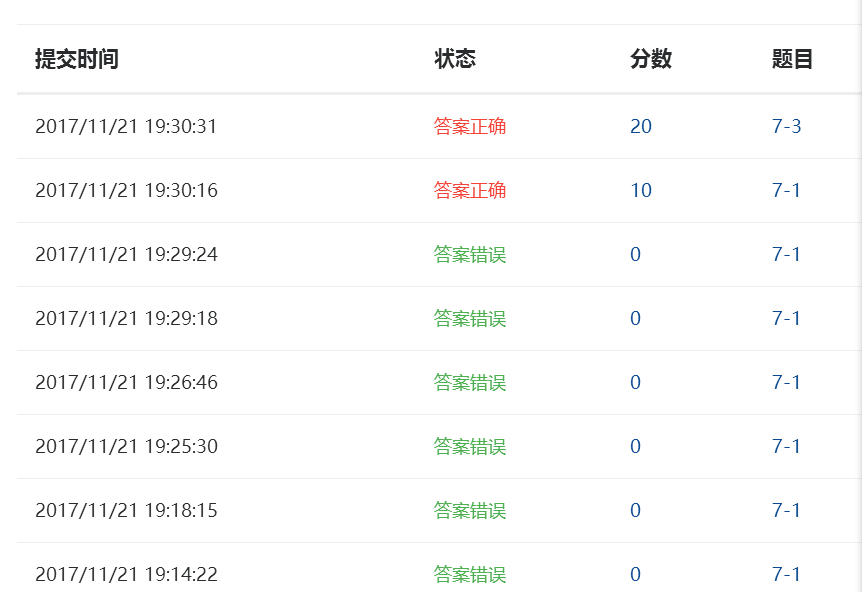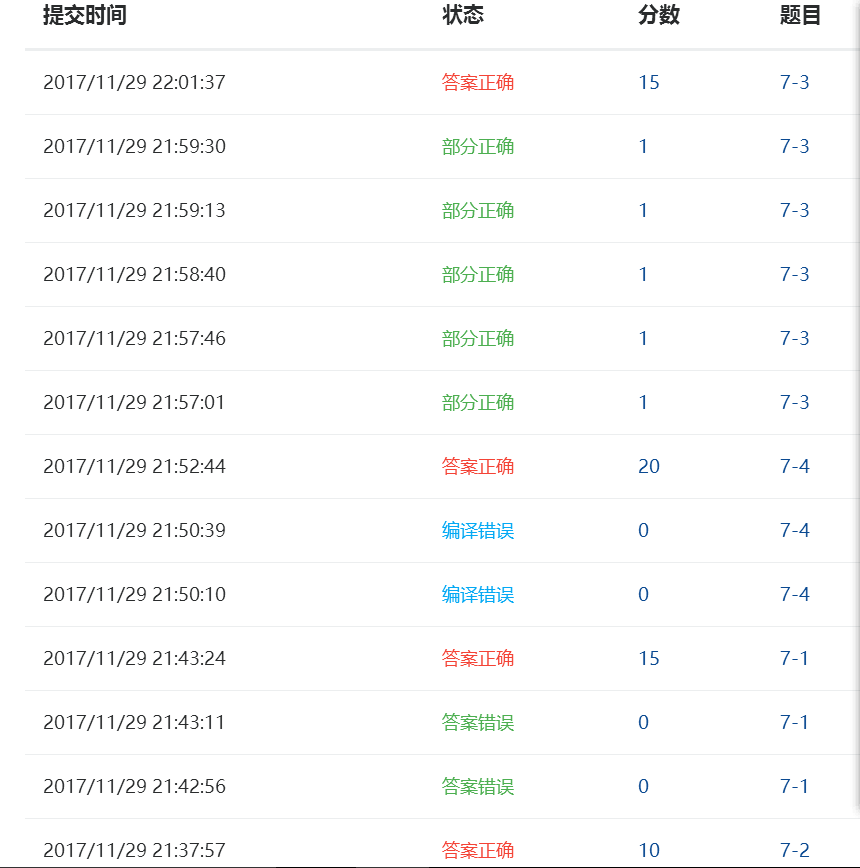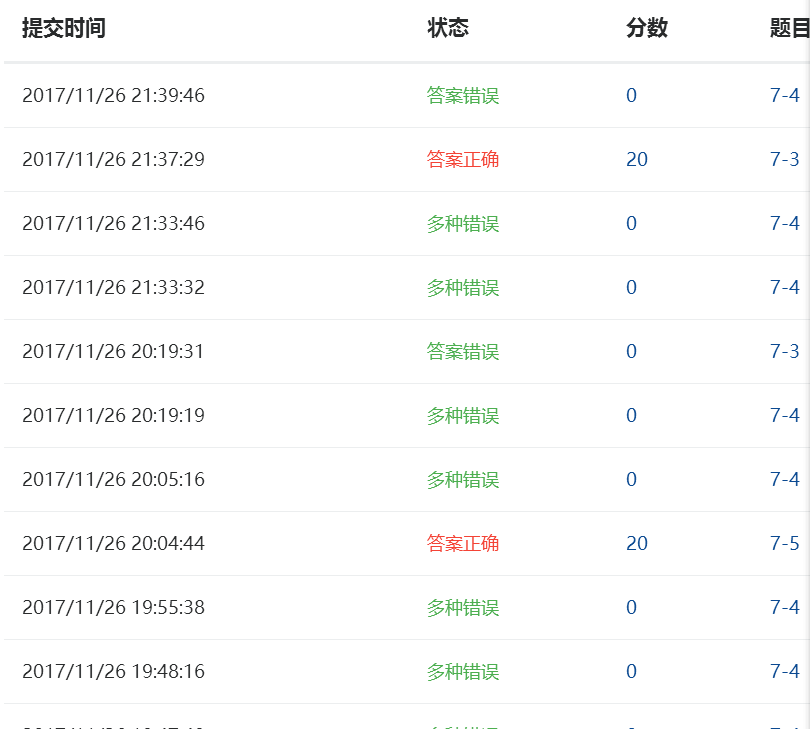（1）我学习到的内容：本周主要学习了数组，先学习了数组的定义，数组元素的引用，数组的初始化，及一些对应的程序。我们从一维数组开始学起,逐渐深入学习了二维数组，还学习了选择法排序及重要的冒泡排序，及一些数组中的数逆序存放，记录下标一些程序。

（2）我认为的难点：选择法和冒泡法不太理解，有点复杂，可能是我没有真正理解，如果理解可能一环套一环很简单吧。

1、点评的同学：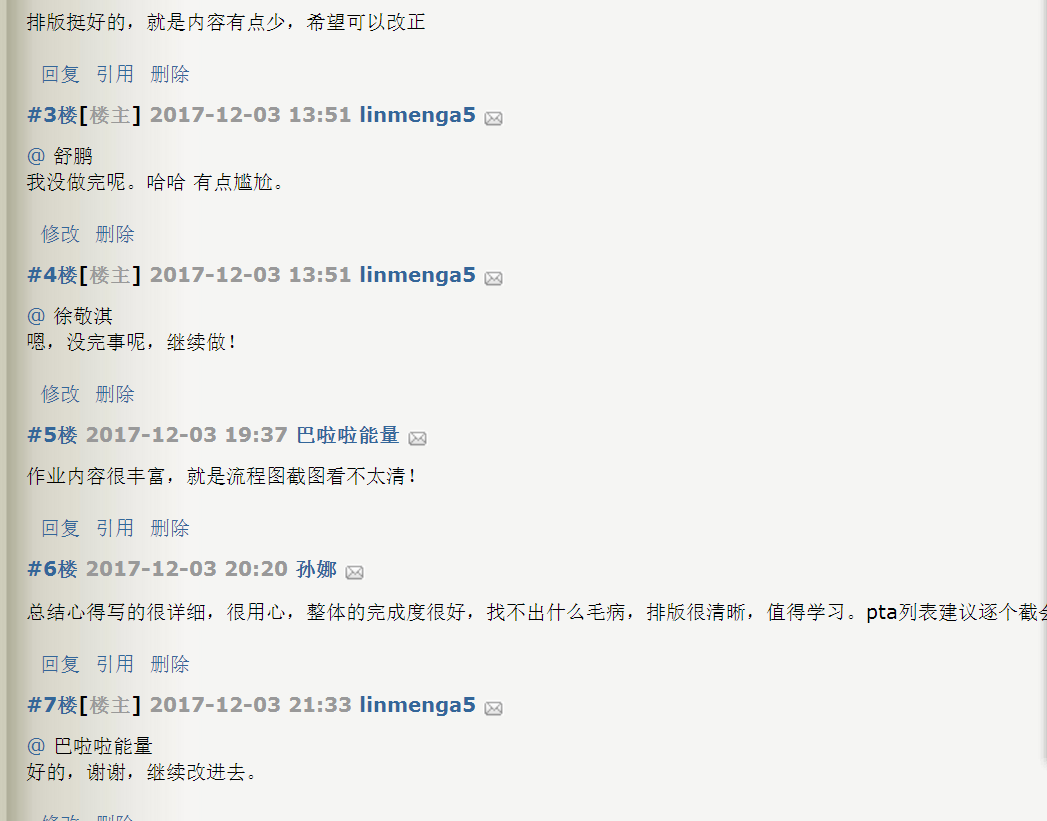2、表格

 时间 代码行数 代码时间 博客字数 博客时间 知识点 11.10 30 15 0 0 for 11.15 35 22 150 15 for,交换，记录下标 11.17 46 35 260 45 选择，冒泡排序 11.19 50 60 300 90 矩阵，循环移动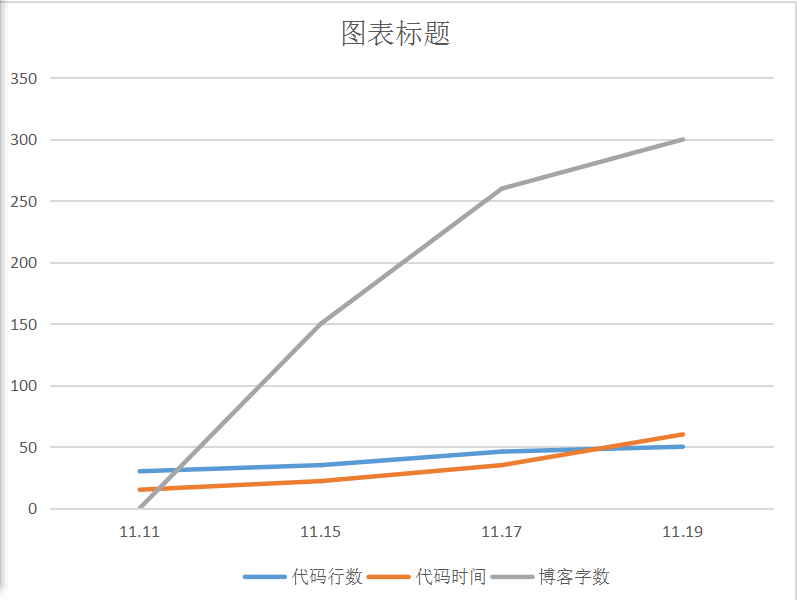posted on 2017-12-02 13:52  linmenga5  阅读(272)  评论(10编辑  收藏  举报